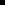### Floating Point Numbers vs. Integers

 Overview Guidelines Example

The active measurement system always uses floating-point numbers for values of points that have conversion to that system. When you use Measurement Units conversion, internal calculations respond to whether you define a point as an integer or a floating point.

The key to deciding whether to define a point as an integer or floating point is to determine how much precision that point requires. A floating point gives you the highest degree of precision.

Here is a brief description of the process.

You define a point as an integer type and assign it a base measurement unit. At runtime the active measurement system causes that base measurement unit to convert to its equivalent unit.

When you set the point's value (a setpoint), the point's value is reverse converted from the active measurement unit into the base measurement unit. If the point is:An integer, in order to fit the reverse converted value into the integer specification, the conversion process rounds off the floating value that was the result of the reverse conversion to the nearest integer.A floating point, it will be able to hold the floating value that was the result of the reverse conversion.

When the point is displayed, the value is forward converted from the base measurement unit into the active measurement unit. If the point is:An integer, the forward conversion will use the rounded value in its calculations. The displayed value will reflect that rounding.A floating point will hold the floating value that was the result of the reverse conversion. The displayed value will equal the value at which you set the point.

In many cases, the rounding effect is totally acceptable. When it is not, use floating-point types.

For points that need to have the highest level of precision and use Measurement Units conversion, use the floating point type instead of the integer point type.

Point XYZ is a point with a base unit ID of inches that requires the highest level of precision. Its initial value is 10 inches.

You use Metric as the active measurement system. As a result, XYZ's value displays as centimeters.

You want to perform a setpoint on XYZ.

Don't

Define XYZ as an integer.

If you then perform a setpoint on XYZ and set it to 26.924 cm.:26.924 cm. is reverse converted to 10.60 inches.Because XYZ is an integer, 10.60 is rounded up to 11 inches.11 inches is forward converted to 27.94 cm.The point value displays as 27.94 cm.

Do

Define XYZ as a floating-point type.

When you then perform a setpoint on XYZ and set it to 26.924 cm., the internal conversion is able to store 10.6 inches. As a result, the point, which is the same value it was set to, displays as 26.924 cm.

 Some guidelines for measurement units.